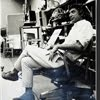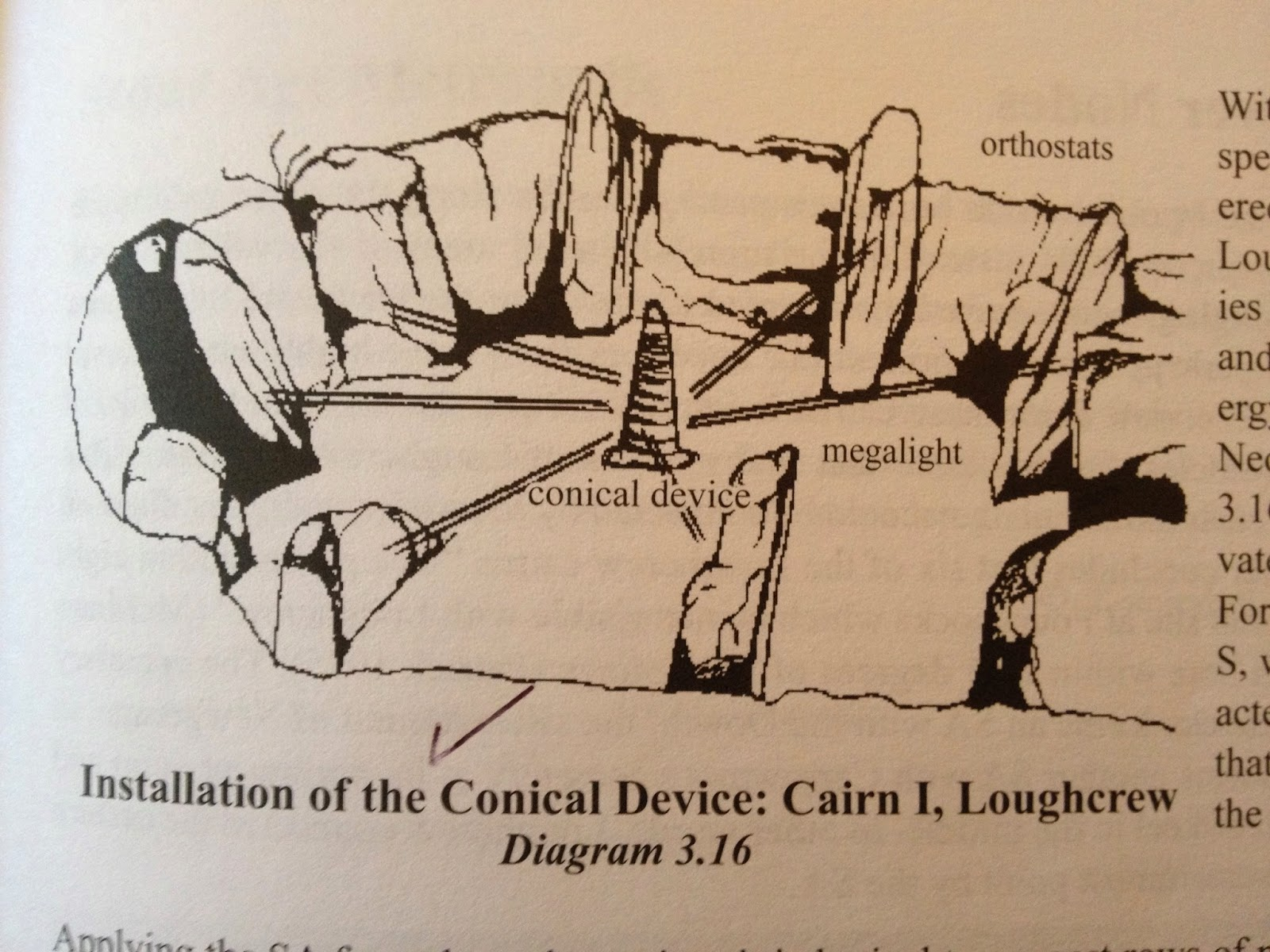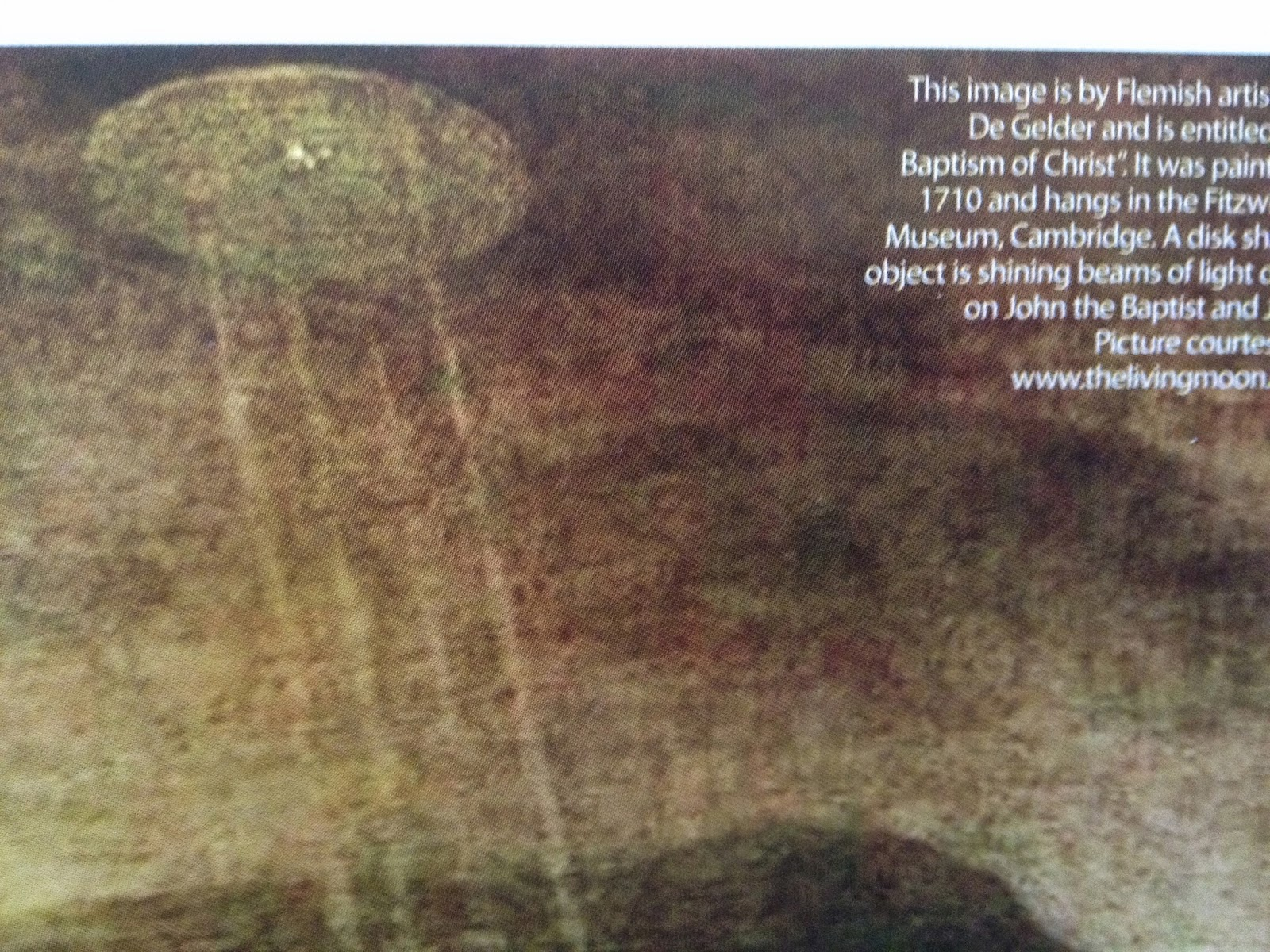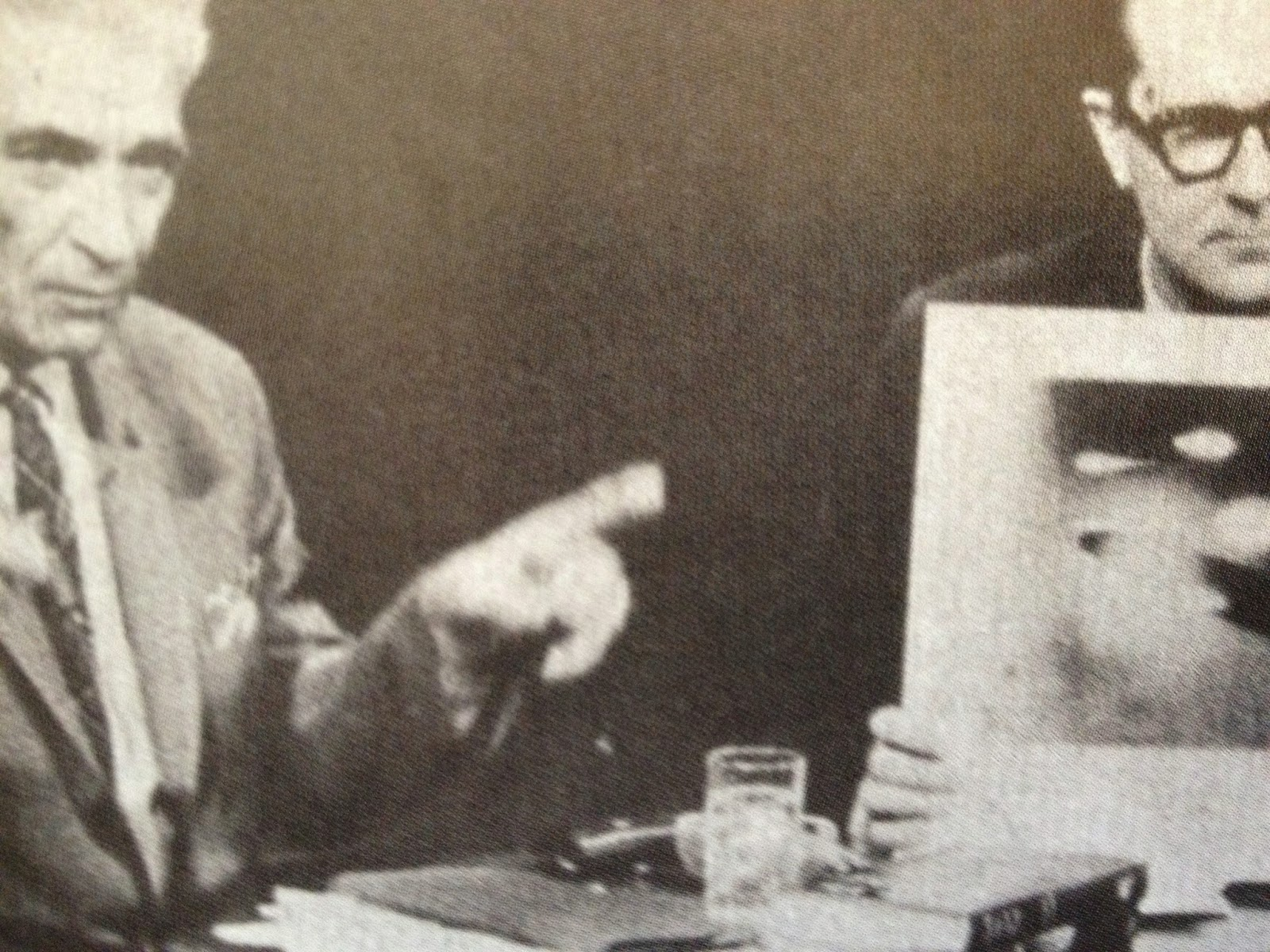## Eugene Ray SDSU 1970'sEugene Ray 1970's. photo credit: Tom Davis

## Tuesday, September 30, 2014

### APOCALYPSE @ SDSU / silver ship biotronic concepts

+FLASH OF LIGHT IN+
>(sdsu /  1969-1996<
>our years of effort)<
+THE EVENING SKY+
^^^^^^^^^^^^^^
>(if you like synchronicity, our silver ship<
+(SILVER SHIP STUDIO+
+/>1699 NAUTILUS ST.)+
>address in lajolla was 1699 nautilus street)<
^^^^^^^^^^^^^^^^^^^^^^^^^^^
+THE NAUTILUS SHELL HAS+
+TO BE OUR PROFOUNDEST+
>(biomorphic structure<
>at its very strongest)<
+LESSON FOR STRUCTURES+
^^^^^^^^^^^^^^^^^^^^^
>(our exhibitions of 1995 and 1975<
>shown in tonight's photo lexicon)<
^^^^^^^^^^^^^^^^^^^^^
>it was my pleasure to design one of our<
>last sdsu exhibitions using econo logs<
+(SDSU / ED 1995)+
>waste plywood cores bought for \$5<
>(commonly known as peeler logs)<
^^^^^^^^^^^^^^^^^^^^^^^^^
>we used these to hang our house models<
>exhibited on circular composite wood<
+(SDSU / ED 1995)+
>chip precut rounds 16 inch diameter<
^^^^^^^^^^^^^^^^^^^^^^^^^
>in the early 1990s our design research was<
>successful in all california competitions &<
+FRANK LLOYD WRIGHT)+
>this exhibition was one of our very best<
>(my taliesin west lecture @ this time)<
^^^^^^^^^^^^^^^^^^^^^^^^^^^^>in the mid 1970s our work w/ pneumatic<
>structures was strong & dave fobes air<
+(SDSU / ED 1975)+
>brush renderings are seen featured<
^^^^^^^^^^^^^^^^^^^^^^^^^
>in the mid 1970s i installed a comprehensive<
>exhibition of our advanced structures with<
+(SDSU / ED 1975)+
>light weight tensile & biomorphics special<
>w/ biotronic struct. domes, rammed earth<
^^^^^^^^^^^^^^^^^^^^^^^^^^^^
>i wrote titled "environ media"…collage was<
+(SDSU / ED 1975)+
>a technique i had started in new orleans &<
>perfected in calif w/ video/slide lectures<
^^^^^^^^^^^^^^^^^^^^^^^^^^^
>my goal was teaching advanced design<
>concepts expressed in graphics that had<
+(SDSU / ED 1975)+
^^^^^^^^^^^^^^^^^^^^^^
>in the mid 1970s our research & development<
>had reached a vital plateau seen in this solar<
+(SDSU / ED 1975)+
>heated residential prototype exhibit above<
^^^^^^^^^^^^^^^^^^^^^^^^^^^^^^
>this vignette in our sdsu studio classroom 301<
+(SDSU / ED 1973)+
>angeles who sent a crew to document<
>my work w/ radiant color and light<
^^^^^^^^^^^^^^^^^^^^^^^
>so the cbs television station in los angeles<
>sent a crew down to document our work<
+BIO- INVENTIONS '73)+
>but they brought lights that ruined our<
^^^^^^^^^^^^^^^^^^^^^^^^
>grad student kotaro nakamura aided me<
>in a regional shopping center concept<
+/ "DESERT INCIDENTS")+
>that i evolved into "desert incidents"<
>this shall be the main design i leave<
^^^^^^^^^^^^^^^^^^^^^^
>in new orleans i had invented a technique<
>collaging fluorescent color paper on the<
>design graphics to express radiance as<
+PHOTO TECHNIQUE)+
>photographed w/ ulta violet light and<
>using infra-red film in my leica 3-f<
^^^^^^^^^^^^^^^^^^^^^^^^^
>i am very grateful to jim bourke who took<
>this photo & alice anda my last student<
>assistant who aided me when i retired<
+(GRATITUDE : MY STUDENT+
+ASSIST'S THRU THE YEARS)+
>after contracting malaria in brazil after<
>my last international exhibition/lecture<
^^^^^^^^^^^^^^^^^^^^^^^^^^

+"DESERT INCIDENTS" & DJED DEVICE+
+OFFERED IN CA. PALM DESERT AREA+
>(ufo/et back engineered technology<
+TO PROVIDE OUR DESIGN CONCEPTS+
^^^^^^^^^^^^^^^^^^^^^^^^^^^^^^^^
eugene ray, mfa, architect
professor emeritus, sdsu

## Monday, September 29, 2014

### BIOTRONIC SYNERGY / research of wun chok bong & myself

+GRATITUDE TO WUN CHOK BONG+
+WHOSE ENERGY RESEARCH HAS+
>("the gods machines"<
>published in 2008)<
+LIGHTED MY OWN RESEARCH+
^^^^^^^^^^^^^^^^^^^^^^^^^^^^
>this was enormous boost to<
>my long task to reveal the<
>wisdom of>biotronics<in<
>architectural volume use<
^^^^^^^^^^^^^^^
+UNIV. OF NANCY, FRANCE+
>was the locus of one of the happiest honors of <
>life when i was invited to join the french govt.<
+(BIOTRONIC ARCHITECTURE+
+RESEARCH PUBLISHED,1986)+
>international architecture & energy comm<
>and they published my biotronic research<
^^^^^^^^^^^^^^^^^^^^^^^^^

+TONIGHT'S BLOG PRESENTS+
+SYNERGY IN THE WORK OF+
>(also david childress<
>& christopher dunn)<
+W.C. BONG AND MYSELF+
^^^^^^^^^^^^^^^^^^^
>i am very pleased that wun chok bong's<
>monumental book has apparently sold<
+(GRATITUDE FOR /+
+HIS DEDICATION)+
>many copies and encouraged his most<
>current volumes on gothic architecture<
^^^^^^^^^^^^^^^^^^^^^^^^^
>ancient stonehenge and its use of vertical<
>stones to construct a biotronic radiance<
+(STONEHENGE: ANCIENT+
+BIOTRONIC ARCHTYPE)+
>in a circular network is an initial study<
>in"the god's machines"profound data<
^^^^^^^^^^^^^^^^^^^^^^^>vertical conical stone energy device is<
>a primary focal of biotronics in "the<
+(STONE CONOID+
>god's machines" polemic lexicon<
^^^^^^^^^^^^^^^^^^^^^^
>this important illustration in "the god's machines"<
>reveals the "djed device" as the source of energy<
+(ANCIENT EGYPT+
+/ ET INTERFACE)+
>for recharging aerial craft of an et construction<
^^^^^^^^^^^^^^^^^^^^^^^^^^^^^^^^^
>it is a profound revelation that wun chok<
>bong, david childress and christopher<
+(BONG, CHILDRESS &+
+DUNN SYNERGETIC)+
>dunn have all concluded that  >many<
>ancient structures are energy sources<
^^^^^^^^^^^^^^^^^^^^^^^
>someday the djed pillar device shall draw<
>many thousands of visitors to the calif<
+/ "DESERT INCIDENTS"+
>desert museum i entitled"desert incidents"<
^^^^^^^^^^^^^^^^^^^^^^^^^^
>(my hope is that a core group shall lead w/<
>dave, jim, ed, kevin, ralph, tom (see note)<
>mary lynn, alice, sherry, jasmin, nancie<
>andrea + others who show lead effort)<
^^^^^^^^^^^^^^^^^^^^^^^^^^
>the djed pillar device is a fascinating reflection<
>of the synergetic interface of egyptian culture<
+(DESERT INCIDENTS UFO-ET+
+MUSEUM FOR CA. DESERT)+
>with extraterrestrial technology and could be<
>a primary factor in my vision of a museum<
^^^^^^^^^^^^^^^^^^^^^^^^^^^^
>the historical powerhouse conoid of mycenae<
>shall again display its biotronic radiance as<
+PYRAMID POWER, images)+
>we recall philip corso's back engineering<
>history that has galvanized paul helyer<
^^^^^^^^^^^^^^^^^^^^^^^
>the energy flow in this diagram is crucial to<
>the vital bio-radiance of an ancient egyptian<
+(EARTH ENERGY UP (right) /+
+RECHARGE(center),WASTE+
+>DISCHARGE DOWN (left))+
>megalithic power generator, ca 3000 bc of<
>"the gods machines"(tesla's inspiration)<
^^^^^^^^^^^^^^^^^^^^^^^^^>the conical roofed prototypes illustrated here<
>are the most profound of all my residential<
+(BIOTRONIC ENERGY /+
+TEMPLAR MEMORY)+
>prototypes regarding use of biotronics &<
>remind us of templar "genetic memory"<
^^^^^^^^^^^^^^^^^^^^^^^^^
>it was my brilliant math wizard friend ron<
>schafer who gave me the formula that i<
+/>> PEOPLE WASH)+
>based this "people wash" prototype on<
>that has ancestry back to ancient egypt<
^^^^^^^^^^^^^^^^^^^^^^^^^
>earlier for the sushi gallery i designed this<
>portable home size "people wash" with<
+/>>WILHELM REICH)+
>a double power (cone & dome) and a<
>memory of wilhelm reich's "orgone"
^^^^^^^^^^^^^^^^^^^^^^^
>i am very grateful for recent kind message<
>to me on Facebook by many of you good<
+(BIOTRONIC ENERGY UNIT+
>friends responding to wun chok bong's<
>dedication to me in his "gothic" books<
^^^^^^^^^^^^^^^^^^^^^^^^^^
*+NOTE : i am also grateful to many alum<
>and friends on the distaff side who have<
+(MARY LYNN=ALICE=SHERRY+
+TANIA=JASMIN=CHIKAKO+
+NANCIE=ANDREA= etc, etc)+
>shown me much aid & good will thru<
>over fifty years of bioenergy research<
^^^^^^^^^^^^^^^^^^^^^^^^
+AND MY BELOVED WIVES+
>for all my beloved family)<
+>MARIAN & MARIANNE<+ !
^^^^^^^^^^^^^^^^^^
eugene ray, mfa, architect
professor emeritus, sdsu

## Sunday, September 28, 2014

### UFO MATRIX / visuals introduce transcendental dimensions

+DRAWINGS, PAINTINGS, PHOTOS+
>in a fine british ufo magazine no longer available in<
>san diego, provide us tonight w/ several important<
+("UFO MATRIX" MAGAZINE+
+TRANSCENDENT ILLUST)+
>topics of transcendental dimension exploration<
>triggered by very talented artists illustrations<
^^^^^^^^^^^^^^^^^^^^^^^^^^^^

+ARTIST'S VISUALS OF UFOS+
+TONIGHT ARE VERY GOOD+
>(artists visuals are necessary<
>when actual photos are not)<,
+REFLECTIONS OF REALITY+
^^^^^^^^^^^^^^^^^^^^^^^^
>my opinion is based on not only having<
>seen a UFO in 1947 but having seen<
+("OPEN MINDS" CONF /+
+ARIZONA in FEB 2015)+
>many excellent videos and photos ln<
conferences, books and magazines<
^^^^^^^^^^^^^^^^^^^^^^^
>i consider my own ufo experience the most<
>profound experience of my life & know<
+>>/ UFO SIGHTING+
>it directed much of my later life & work<
>(the craft i saw was a huge mothership)<
^^^^^^^^^^^^^^^^^^^^^^^^^^>this excellent graphic illustrates the power<
>of the crafts radiant energy and movement<
+>>TIMOTHY GOOD)+
>reninding us of the biotronic recharging<
^^^^^^^^^^^^^^^^^^^^^^^^^^^
>the better type of average persons interpretation<
>of the classic "flying saucer" sighting, although<
+>>RICHARD DOLAN)+
>in this case the saucer is not domical at the top<
^^^^^^^^^^^^^^^^^^^^^^^^^^^^
>"haunted skys" the encyclopedia of british<
>UFO data has this graphic on the cover<
+>>JOHN MACK, Ph.D)+
>expressing the great variety of types<
^^^^^^^^^^^^^^^^^^^^^
>the illustrations in "ufo matrix" are particularly<
>good, as is this issue (volume1issue5) which is<
+JAMES MCDONALD PhD)+
>impressive w/ the variety of its feature articles<
^^^^^^^^^^^^^^^^^^^^^^^^^^^^^^>this "transcendental manifestation" painting<
>of a ufo was made in 1710 forty nine years<
+>>TEMPLAR DOMES+
>before our first gruy merovingian ancestors<
>arrived in new orleans w/ "templar vision"<
^^^^^^^^^^^^^^^^^^^^^^^^^^
>this famous and fantastic flemish painting by<
>aert de gelder of a UFO sending energy rays<
+/>de GRUY / BELGIUM)+
>down to jean de baptiste & jesus is special<
>to our degruy who had a flemish branch<
^^^^^^^^^^^^^^^^^^^^^^^^^^^>this very important photo of UFO history has<
>george adamski left, pointing out details to<
>long john NEBEL on his famed radio show<
>(1950 n.y.) of his most dramatic photograph<
^^^^^^^^^^^^^^^^^^^^^^^^^^^
>i feel a kindred spirit w/ erich von daniken<
>not just because he has a swiss heritage<
+DJED PILLAR DEVICE)+
>but because he found the UFO truth<
^^^^^^^^^^^^^^^^^^^^^^^^^^
>like christopher dunn, von daniken knows<
+>CHRISTOPHER DUNN)+
>could finance our DJED PILLAR)<
^^^^^^^^^^^^^^^^^^^^^^^
>this museum installation is from the "mystery<
>park" in switzerland of Erich von Daniken<
+>>ERICH von DANKEN)+
>illustrating egyptian stone drilling with<
>modern drills of ancient alien design<
^^^^^^^^^^^^^^^^^^^^^^^^
>accolades must go to UFO research groups<
>like the brisbane based queensland group<
+UFO REPORTING CENTER)+
>in australia and peter davenport of the<
>UFO reporting center based in wash.<
^^^^^^^^^^^^^^^^^^^^^
>it is very synchronistic that this illustration of<
>jean de baptiste w/ the holy grail appears in<
+JEAN BAPTISTE de GRUY)+
>this issue of "ufo matrix" considering family<
>de gruy history & devotion to "de baptiste"
^^^^^^^^^^^^^^^^^^^^^^^^^

+JACQUE VALLE, Ph.D SUGGESTS+
+A SPIRITUAL UFO CONNECTION+
>wormhole time travel)<
+ALSO TRAVEL VIA WORMHOLE+
^^^^^^^^^^^^^^^^^^^^^^^^^^
eugene ray, mfa, architect
professor emeritus, sdsu

## Saturday, September 27, 2014

### TENSILE STRUCTURES / nature our best guide

>(resistent to hurricanes<
>>and earth quakes)< !
+IS TENSILE & RESILIENT+
^^^^^^^^^^^^^^^^^^^^^

+LINDA MOULTON HOWE+
>(earth files & earth files . com)<
^^^^^^^^^^^^^^^^^^
>is like our profound new research friend<
+STACE TUSSEL-COLLIGAN+
>(inter-intelligence-communications.com)<
^^^^^^^^^^^^^^^^^^^^^^
+IMPRESSIVE RESEARCHERS+
>of ufo and related phenomena like<
>crop circles and cattle mutiliation<
^^^^^^^^^^^^^^^^^^^^^^
+NON-BELIEVERS+
>should listen to a cattle man on "coast to<
+(SEE STACE'S IMPRESSIVE+
+VIDEO DOCUMENTARIES)+
>his cattle in the middle of a long night<
>"not of this world", (his explanation)<
^^^^^^^^^^^^^^^^^^^^^^^
>this 1969 version of the whole earth catalog was<
>a great reference for early sdsu environ design<
+(ENVIRON DESIGN+
+>>PRIMARY REF)+
>classes that i taught w/ biotronics in mind<
>(stewart brand's book won pulitzer prize)<
^^^^^^^^^^^^^^^^^^^^^^^^^^^^
>you some idea of the organic systems side w/<
+(BIOMORPHIC BONE+
+NATURAL DESIGN)+
>exellent reference to tensile natural structure<
>cosmic consciousness was the driving force<
^^^^^^^^^^^^^^^^^^^^^^^^^^^

>two different uses of tensile structure in my<
>oeuvre from tensile fiber optic cable (top)<
+(TENSILE IS LIGHT WT+
+>THUS ECONOMICAL)+
>to bent structural frame for bungalows<
^^^^^^^^^^^^^^^^^^^^^^^^^^^
>this house near new orleans in framing stage<
>reveals a light, open, tensile structure that<
+>>WILTY RESIDENCE+
>was economical and assembled quickly<
>(built 2 mos, w/ two young carpenters)<
^^^^^^^^^^^^^^^^^^^^^^^^^^>i consider this tensile structure design the key<
>design for future generations to my oeuvre of<
+HEALTHY ECONOMICAL)+
>radiant biotronic architecture, good for health<
>(this concept radiant with conoidal energy)<
^^^^^^^^^^^^^^^^^^^^^^^^^^
>my tensile structure conoid for the san diego<
>native american community combines the<
+(CONOID IS SUPERIOR+
>historical "teepee" with fiber optic cables<
>(light weight structure earns economy)<
^^^^^^^^^^^^^^^^^^^^^^^^^^^^
>there is no doubt that monocoque shells are<
>the strongest enclosure known for human<
+(NATURE IS OUR+
+>>BEST GUIDE)+
>habitation, (ferro-cem is economy tech)<
^^^^^^^^^^^^^^^^^^^^^^^
>tensile worm hole, time travel technology<
>may yet save from earth's negative ways<
+(FOR MY OLD FRIEND+
>(be aware of much progress in this)<
^^^^^^^^^^^^^^^^^^^^^^^^^^^^
>cosmic consciousness is essential to radiant<
>architecture and the research of dedicated<
+(TRANSCENDENTAL+
+MANIFESTATIONS)+ !
>scholars like stace tussel colligan and<
>linda moulton howe very important<
^^^^^^^^^^^^^^^^^^^^^^^^

>tensile structure was essential to my sdsu class<
>"synergetic environments", two different<
+(TENSILE SYNERGY @ +
+70s ENVIRON DESIGN)+
>designs we see from my 1970s archive<
>(cosmic consciousness a strong factor)<
^^^^^^^^^^^^^^^^^^^^^^^^
>robert lazar is just one of many talented physics<
>genius whistle blowers who worked for the<